# How to Copy Cells If Column Contains Specific Value/Text in Excel?

Copying data in Excel is a very involved process, but have you ever tried to copy based on a single element in the sentence? If we need to copy sentences based on a single element manually, it can be a time-consuming process. we can complete this task in very less time by following the tasks. Read this tutorial to learn how you can copy cells if a column contains specific values or text in Excel.

## Copy Cells If a Column Contains Specific Value/Text Using Filter

Here, we will first use the filter option, then use the text filter and copy data in the default way and use the paste special to complete the task. Let us see a simple process to know how we can copy cells if a column contains specific values or texts using a filter in Excel. We can use this process if the data is in table format.

### Step 1

Consider the following Excel sheet, which contains a table similar to the one below.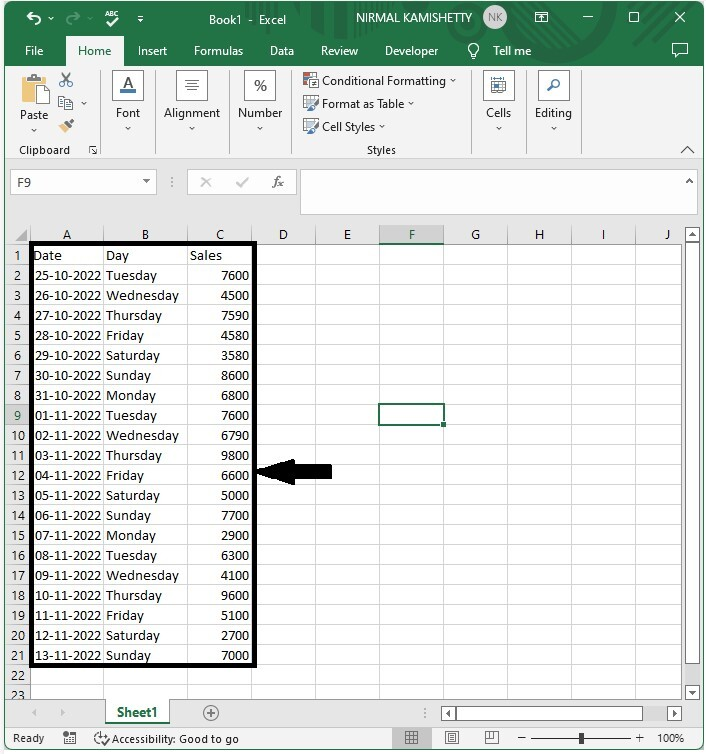Now select the data, then click data, then filter.

Select data > data > filter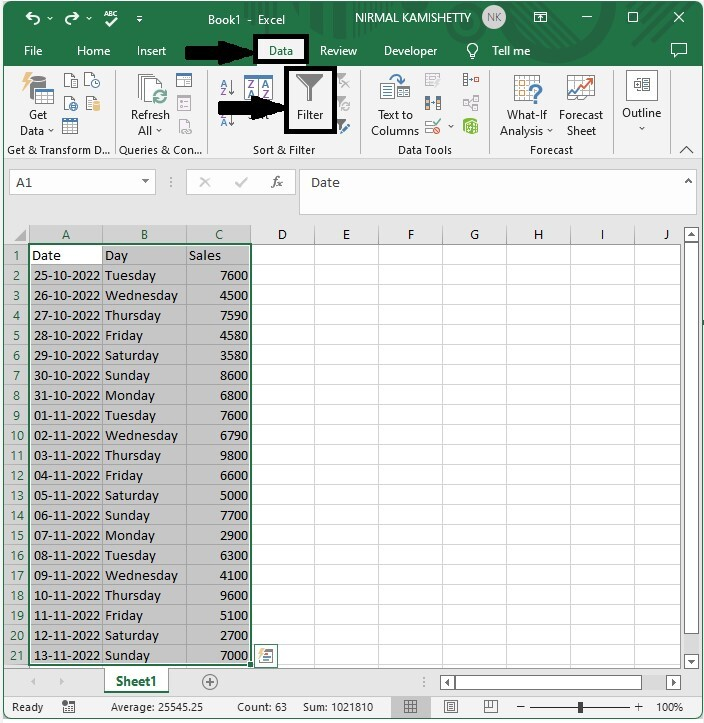### Step 2

Now click on the down arrow, then click on text filers, and select contains from the list as shown in the below image.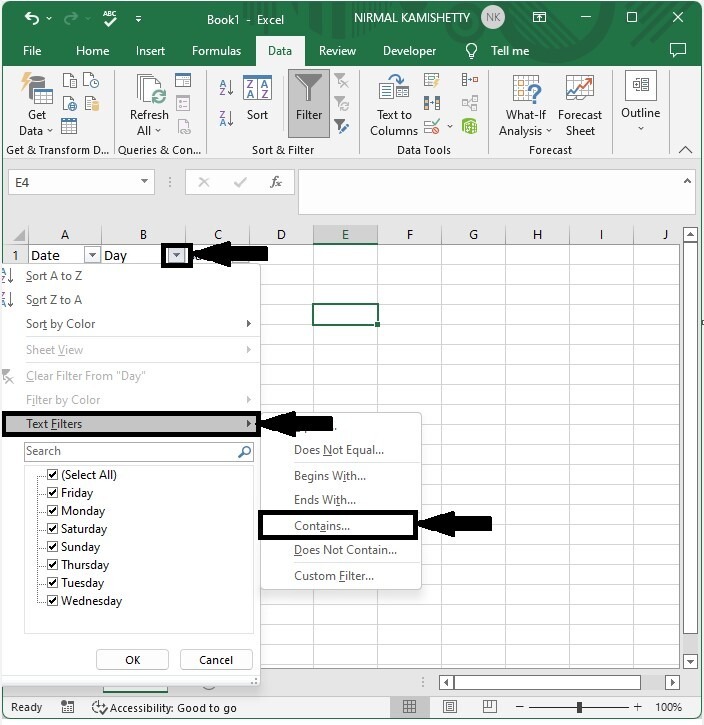### Step 3

Then enter the value you want to copy and click OK.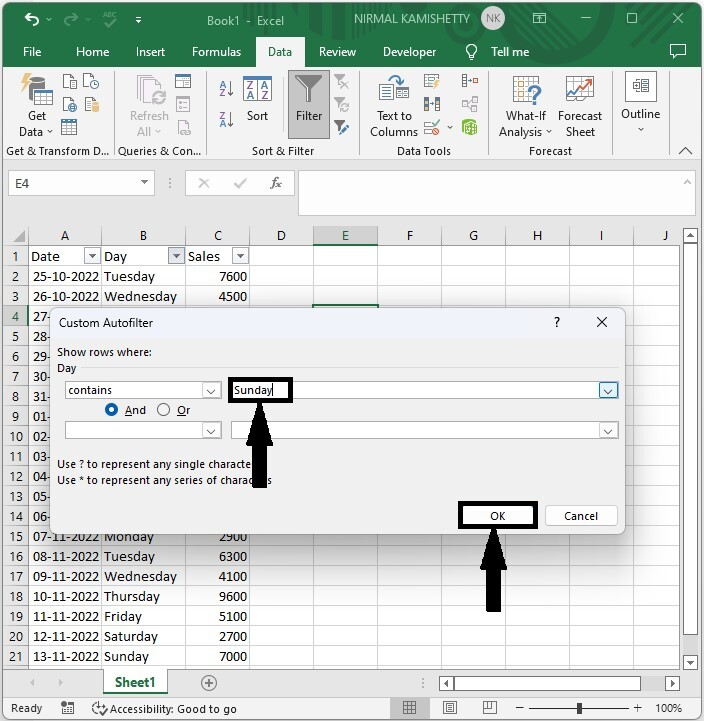### Step 4

Then select the range of cells and use the commands CTRL + C to copy the cells and CTRL + V to paste the values.

## Copy Cells If Column Contains Specific Value/Text Using Find

Here we will use "find and replace" and then copy the cells from there. Let's go over a simple procedure for copying cells in Excel if a column contains specific values or text.

### Step 1

Let us consider an Excel sheet where the data is similar to the below image.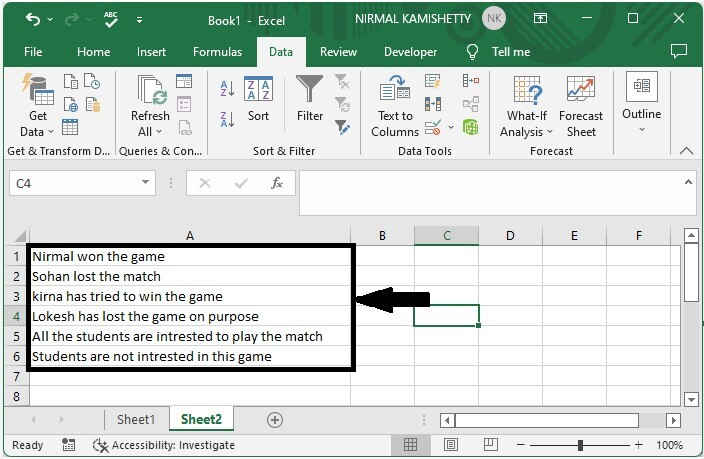Now, press CTRL + F to open find and replace, then enter the value in find what and press find.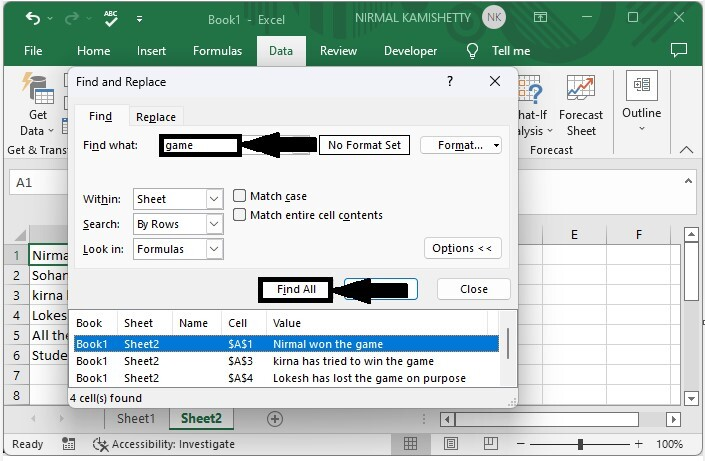### Step 2

Now select all the results and close the pop-up window.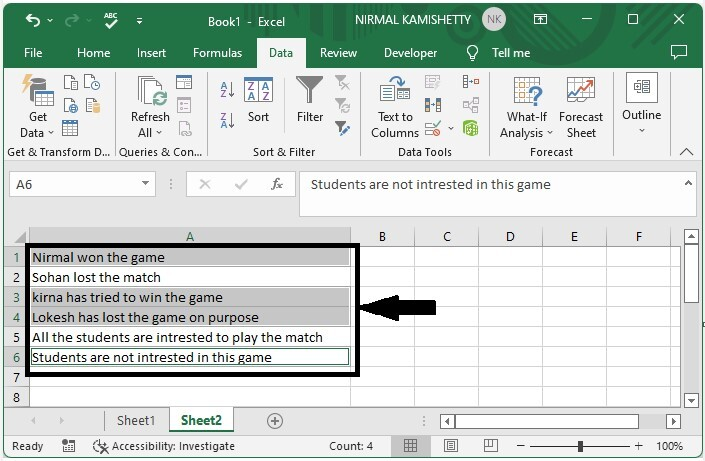### Step 3

Now use the commands CTRL + C to copy the cells and CTRL + V to paste the values.

## Conclusion

In this tutorial, we used a simple example to demonstrate how you can copy cells if a column contains a specific value in Excel.